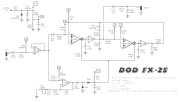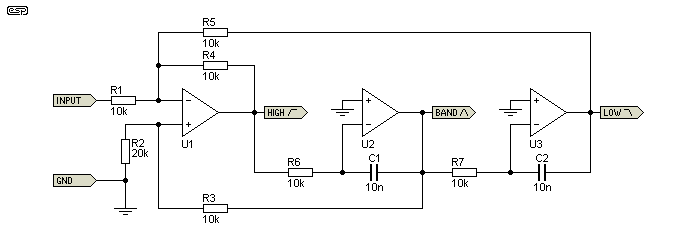###Author Topic: DOD FX25 - How does it work?  (Read 1266 times)

#### bushidov##### DOD FX25 - How does it work?
« on: August 15, 2020, 04:58:30 AM »
So I built me a clone of a DOD FX25 AutoWah the other day using this schematic:Works great, but I am not satisfied just knowing that it works, but also how it works.

I am new to transconductance amplifiers, so I really have no clue what the whole circuit is doing.

I can see I have a power supply and a bias to half voltage. I see I have an opamp (IC1B) as an input buffer, but am not sure what exactly IC1A is doing, other than that effects the sensitivity with a 100K Pot (P1). It looks like a non-inverting op-amp gain stage like I'd find on an overdrive pedal, but the D2/D3 and R9/C7 section isn't something I am familiar with.

I see C11 on out to the output is familiar, but the whole LM13600/LM13700 part is something totally new to me, schematically. So what is it doing? What resistors and capacitors are doing what?

Sorry to keep being a man of a million questions, and thanks for any help!
"A designer knows he has achieved perfection not when there is nothing left to add, but when there is nothing left to take away."

- Antoine de Saint-Exupéry

#### Rob Strand

• more
• Awesome!
••• Posts: 9007
• Total likes: 4021
• ทำเต็มที่##### Re: DOD FX25 - How does it work?
« Reply #1 on: August 15, 2020, 06:27:45 AM »
The simple answer is the output at pin 8 IC2 is a band-pass filter.   The filter cut-off is determined by the current feeding into pins 1 and 16.

The OTA:
So the way the OTA's work is the input is a voltage, the voltage difference between pin 3 (V+)  and pin 4 (V-).   That voltage is multiplied by the trans-conductance gm which produce a current at pin 7, Iout = gm * (V+ - V-)   The current feeds the impedance Z to ground on pin 9 which converts the output current into a voltage (on pin 7),   Vout = gm * (V+ - V-) * Z.   The chip has a buffer with input on pin 7 and output on pin 8.  So the voltage on pin 8 is Vout = gm * (V+ - V-) * Z.    The impedance to ground   Z = 1/sC.    So Vout = (gm / (sC)) * (V+ - V-). The transductance is a proportional to the current going into pin 1,  see datasheet.

The dividers:
pin 3 is fed from a divider 10k + 1k fed from Vin
pin 4 is fed from two dividers 22k + 22k + 1k  which sum and are fed from pin 8 and pin 9
pin 14 is fed from a divider  22k + 1k which is fed from pin 8

Suppose we call the output at pin 8 V1 and the output at pin 9 V2.

In order to simplify things, I'm going to say a divider of 10k  + 1k is multiplying by a factor k (~ 1/10) and that the 22k + 1k divider is about half that so k/2.   So,

V+   = k * Vin
V-     = (k/2) * (V2 + V1)

The aim of the game is to solve the circuit to get the output terminal voltage V1  in terms of Vin.

From the way the OTA works,

V1  = (gm / (sC)) * (V+ - V-)
= (gm / (sC))  * (  k Vin   - (k/2) (V2 + V1) )     ; substituting V+ and V- above

V2  = (gm / (sC)) * (k/2) V1

Next get rid of V2 from the V1 equation,

V1   = k (gm / (sC))  {Vin -  (1/2) *[ (gm / (sC)) * (k/2) V1  + V1 ] }

Shuffle the V1's over to the left hand side,

(sC / (k gm)) * V1   =  Vin -  (1/2) [ (gm / (sC)) * (k/2)  + 1]  V1

{ (sC / (k gm))  +  (1/2)*[ (gm / (sC)) * (k/2)  + 1] } V1 =  Vin

{ (sC / (k gm))   +  (k gm / (4 sC))  + 1/2  }   V1  = Vin

Multiply both sides by (4 sC) / (k gm)

{s^2 (2 C/(k gm))^2  + 1   +  s (2 C) / (k gm)} V1 = Vin s  (2C / (k gm))

Which gives the transfer function,

V1 / Vin          =                                 s  (2 C / (k gm))
----------------------------------------------------------------
s^2 (2 C/(k gm))^2  +   s (2 C) / (k gm)   + 1

=                          ( s/w0)
--------------------------------------------
(s / w0)^2   +  s / (Q w0)   +     1

where w0 = k gm / (2C),   Q = 1

This transfer function is a second order band-pass filter  with center frequency w0  = 2 pi f0, and a Q of 1.

You can look-up in the data sheet how gm is a function of Iabc.   If you work out the range of Iabc  then you can work out the range of gm and then calculate the range of the center frequency.

It's a bit rough but that's the general way to approach it.   I could have stuffed-up here and there above and I haven't tried to verify the f0 equation with a simulation.

It would be worthwhile trying to work through it yourself.

If you wanted to work out what each resistor does you would have to have many k's k1, k2 etc for each divider.  Then you will end-up with a big messy equation similar to what I have done with many k's.  Then you have to write the k's in terms of each of the R's in the divider and you get an even bigger mess.

You would need to do that if you wanted to try to change the Q for example, although you could do that just with the k's in the equation.

« Last Edit: August 15, 2020, 06:56:53 AM by Rob Strand »
Send:     . .- .-. - .... / - --- / --. --- .-. -

#### 11-90-an

• Awesome!
•• Posts: 971
• Total likes: 395
• 11-90-an - PH##### Re: DOD FX25 - How does it work?
« Reply #2 on: August 15, 2020, 06:33:45 AM »
IC1A is a gain stage...Basically, it controlls how big the guitar signal is before being fed into a half wave rectifier, which then controls Iabc on IC2

So essentially, the amplified sound here isnt part of the sound circuit, but simply generates a big enough current to control the OTA
flip flop flip flop flip

#### ElectricDruid

• Awesome!
••• Posts: 5534
• Total likes: 3210
• Tom W.##### Re: DOD FX25 - How does it work?
« Reply #3 on: August 15, 2020, 06:54:22 AM »
Ok, so Rob's given you a lot of detail. I'll fill in some broad-brush stuff.

It's an example of a state-variable filter. There are two common varieties - ones with a mixer on the front that provides a highpass output, and ones without a mixer that don't. This is the second type.

State variable filters are built using integrators. A 12dB/oct filter like this needs two. They don't have to be voltage or current controlled. You can just use a dual-gang pot or plain resistors if you don't need it variable, like this:(From https://sound-au.com/articles/state-variable.htm - worth a read)

In the DOD FX25 the OTAs are used to control the current fed to the caps, like a pot would do.

State variable filters are great because they have different filter responses available at the same time. I mentioned the Highpass output you sometimes see from the first mixer (if it's there) but there is also a bandpass output from the first filter stage (IC2a - used as the output in the FX25) and a lowpass output from the second filter stage (IC2b). Try taking C11 to pin 9 of the LM13600 instead of pin 8 and see how you like the lowpass output.

Compare the FX25 schematic with my FilterFX, which uses a similar state variable filter, but using vactrols in place of OTAs. Mine includes the input mixer, so there's a highpass option too.• SUPPORTER

#### bushidov##### Re: DOD FX25 - How does it work?
« Reply #4 on: June 02, 2021, 06:47:48 PM »
Sorry to come back to this, but I how a lot of this is working now, but am still a little confused what the diodes D2 and D3 are there for. I noticed in a simulation, they went from a regular sine wave form to an almost DC wave form that changes voltage over time (basically, gradually drops)

Any help on explaining what is going on there? It kind of looks like a half-bridge rectifier?
« Last Edit: June 02, 2021, 06:51:24 PM by bushidov »
"A designer knows he has achieved perfection not when there is nothing left to add, but when there is nothing left to take away."

- Antoine de Saint-Exupéry

#### bushidov##### Re: DOD FX25 - How does it work?
« Reply #5 on: June 02, 2021, 06:54:28 PM »